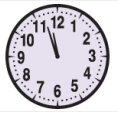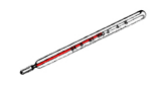### Factors, Multiples and Estimation - Worksheets

CBSE Worksheet-01

Class - IV Mathematics – Factors, Multiples and Estimation

Time Allowed: 30 minutes

1. Fill in the blanks with suitable words:
1. 9,623 round to the nearest hundreds place will yield the answer as ______________.

2. 28, 42, 63, and 70 are all multiples of _____.

3. A ________ multiple is a number that is a multiple of two or more numbers.

4.The time shown here is 11:56. If rounded, then it will be ___________.

5. A number is a ______number if it has more than two factors.

2. State whether each of the following statement is True or False:
1.This thermometer reading is 87.4o. It will be 90o, when rounded to the nearest 10.

2. 4 is a common factor of 12 and 16.

3. 5 is a multiple of 20.

4. 18, 24, and 30 are all multiples of three.

5. All prime numbers are odd numbers.

3. Estimate the product to the nearest hundred. 378 $×$ 119

1. 50000

2. 40000

3. 45000

4. 44982

4. Round off the given whole number to the nearest tens: 906

1. 905

2. 900

3. 910

4. 1000

5. Estimate the difference by rounding each number to the nearest ten. 6887 - 4774 = ___________

1. 2100

2. 2110

3. 2113

4. 2120

6. Estimate the difference to the nearest hundred 85093-41397

1. 43696

2. 43600

3. 43650

4. 43700

7. Estimate the difference to the nearest thousand. 46005-39466

1. 6539

2. 7000

3. 6600

4. 6000

8. List the numbers that have 30 as a multiple.

9. Write all the Prime numbers between 45 and 85.

10. Find the following numbers between 1 to 30.

1. Even numbers
2. Odd numbers
11. Test whether 72148 is divisible by 8 or not?

12. Find five multiples of the following numbers.

1. 25
2. 22
13. Find 3 common multiples of 10 and 15.

14. Do as directed:

1. Estimate the product to the nearest 100:
1. 78 $×$ 22
2. 24 $×$ 14
2. Estimate the division to the nearest 1000:
1. 8380 $÷$ 3812
2. 9440 $÷$ 2886
15. Write the missing multiple of the given numbers

 1 2 3 4 5 6 MULTIPLES OF 11 11 22 55 MULTIPLES OF 16 16 64 MULTIPLES OF 10 10 40 50 MULTIPLES OF 20 20 60 120
1. Find the least number which is divided by 25, 50, and 75 exactly.
2. Find the greatest number which divides 25, 50, and 75 exactly.

CBSE Worksheet-01
Class - IV Mathematics – Factors, Multiples and Estimation

Solution

1. Fill in the blanks with suitable words:

1. 9600. As the number 23 is less than 50 so the rounding off will be done to the lower end.

2. 7.
$×$ 4 = 28, 7 $×$ 6 = 42, 7 $×$ 9 = 63, 7 $×$ 10 = 70

3. Common. Like 5 is the common multiple of 10 and 50.

4. 12:00. We’re much more likely to say “it’s just before 12 o’clock” or “it’s nearly 12”.

5. Composite number. A number with more than two factors is called a composite number.

2. State whether each of the following statement is True or False:

1. True, We can round this to 87° (to the nearest whole number) or to 90° (to the nearest ten).

2. True, 4 is a common factor of 12 and 16.

3. False. 5 is a factor of 20, not a multiple of 20.

4. True. 3 × 6 = 18, 3 × 8 = 24 and 3 × 10 = 30.

5. False.
2 is a prime number which is even.

3. (b) 40000
Explanation:
Round off of 378(400) and 119(100).
Product = 400*100= 40000.
4. (c) 910
Explanation:
We need to round off to the nearest tens than we have to take only last digit that is 6. 6 is greater than 5 so we need to move forward and we will get 910.
5. (d) 2120
Explanation: 6890 - 4770 = 2120
6. (d) 43700
Explanation: 85093-41397= 43696
So, the nearest hundred of 43696 is 43700.
7. (b) 7000
Explanation:
Round off of 46005 & 39466 to nearest thousand is 46000 & 39000 respectively.
Difference= 46000-39000=7000.
8. 1, 2, 3, 5, 6, 10, 30

9. 47, 53, 59, 61, 67, 71, 73, 79, 83 are the prime numbers between 45 and 85.

1. Even numbers
2, 4, 6, 8, 10, 12, 14, 16, 18, 20, 22, 24, 26, 28
2. Odd numbers
3, 5, 7, 9, 11, 13, 15, 17, 19, 21, 23, 25, 27, 29
10. Here, the number formed by the last 3 digits is 148, which is not divisible by 8.
So, 72148 is not divisible by 8.

1. 25
25 $×$ 1=25
25 $×$ 2=50
25 $×$ 3=75
25 $×$ 4=100
25 $×$ 5=125
25, 50, 75, 100, 125
2. 22
22 $×$ 1=22
22 $×$ 2=44
22 $×$ 3=66
22 $×$ 4=88
22 $×$ 5=110
22, 44, 66, 88, 110
• Multiples of 10
10, 20, 30, 40, 50, 60, 70, 80, 90.....
• Multiples of 15
15, 30, 45, 60, 75, 90........
3 common multiples 0f 10 and 15 = 30, 60, 90
1. Estimate the product to the nearest 10:
1. 78 $×$ 22
Round off 78 to the nearest 10 = 80
Round off 22 to the nearest 10 = 20
80 $×$ 20 = 1600
2. 24 $×$ 14
Round off 24 to the nearest 10 = 20
Round off 14 to the nearest 10 = 10
20 $×$ 10 = 200
2. Estimate division to the nearest 1000:
1. 8380 $÷$ 3812
Round off 8380 to the nearest 1000 = 8000
Round off 3812 to the nearest 1000 = 4000
8000 $÷$ 4000 = 2
2. 9440 $÷$ 2886
Round off 9440 to the nearest 1000 = 9000
Round off 2886 to the nearest 1000 = 3000
9000 $÷$ 3000 = 3
11.  1 2 3 4 5 6 MULTIPLES OF 11 11 22 33 44 55 66 MULTIPLES OF 16 16 32 48 64 80 96 MULTIPLES OF 10 10 20 30 40 50 60 MULTIPLES OF 20 20 40 60 80 100 120
1. The least number which is divided by 25, 50 and 75 exactly
L.C.M. of 25, 50 and 75
Multiple of 25 = 25, 50, 75, 100, 125, 150.....
Multiple of 50 = 50, 100, 150, 200, 250...
Multiple of 75 = 75, 150, 225, 300...
L.C.M = 150
The least number which is divided by 25, 50, and 75 exactly is 150.
2. The greatest number which divides 25,50 and 75 exactly
H.C.F. of 25, 50 and 75
Factors of 25 = 1, 5, 25
Factors of 50 = 1, 2, 5, 10, 25, 50
Factors of 75 = 1, 3, 5, 15, 25, 75
H.C.F = 25
The greatest number which divides 25, 50, and 75 exactly is 25.